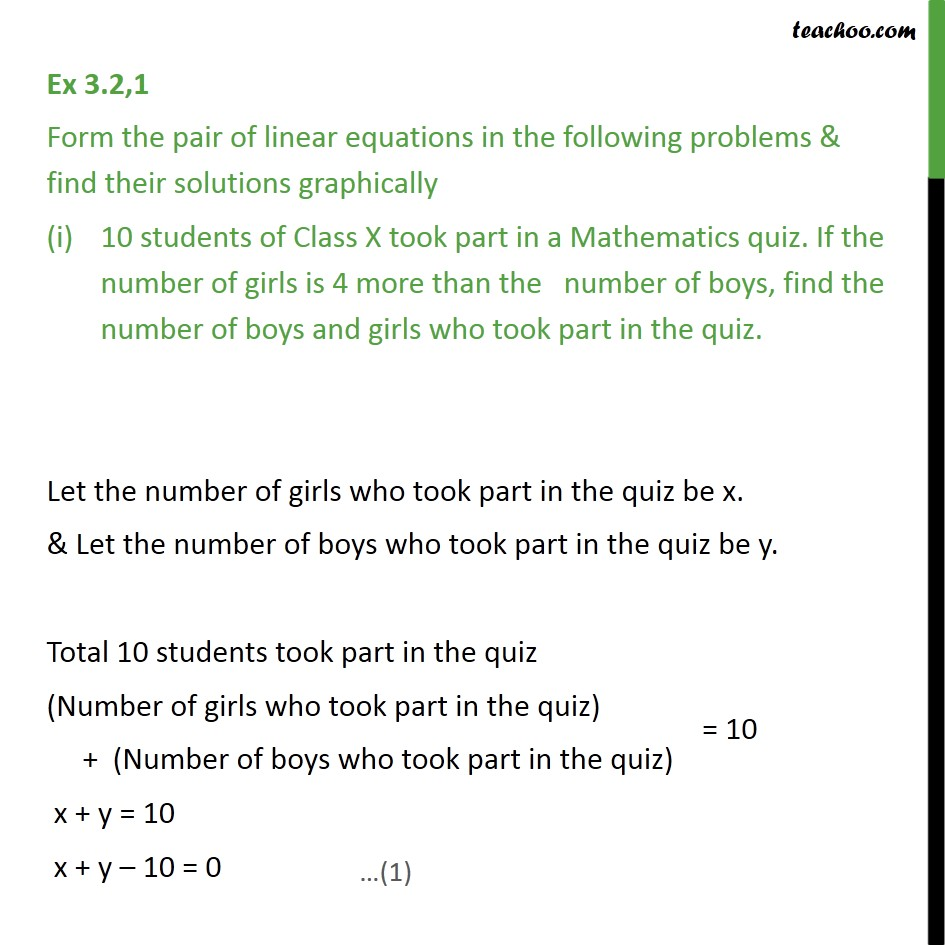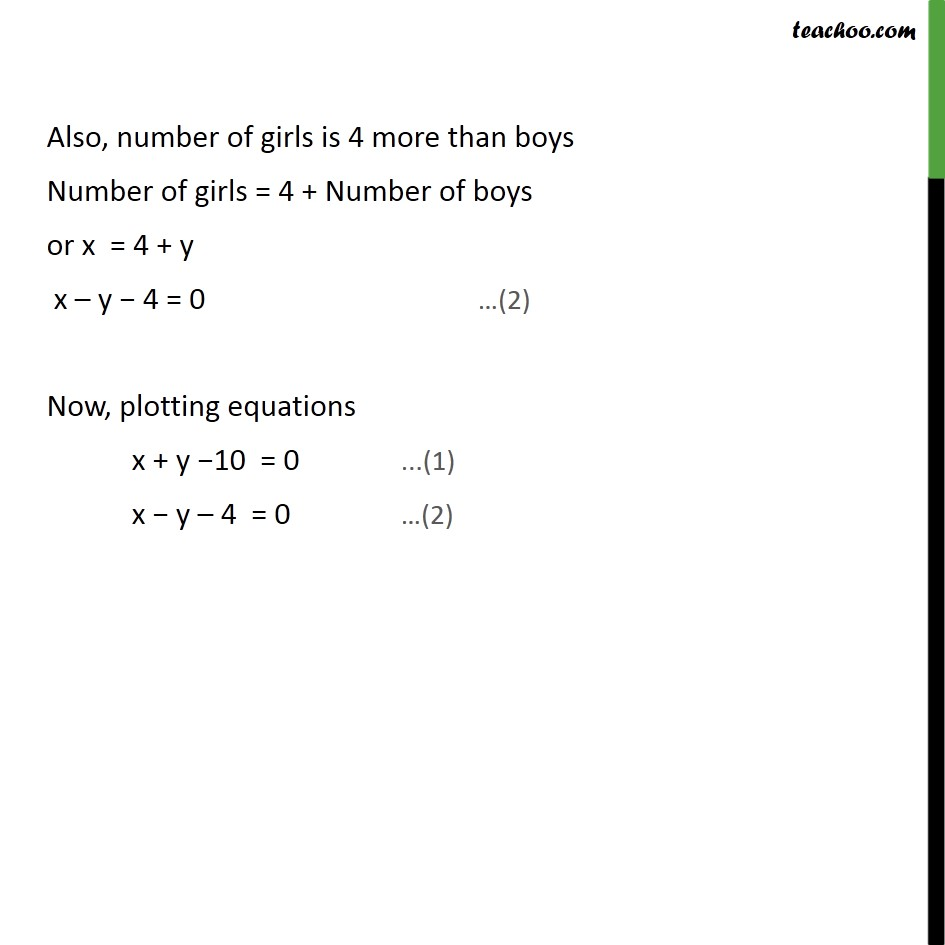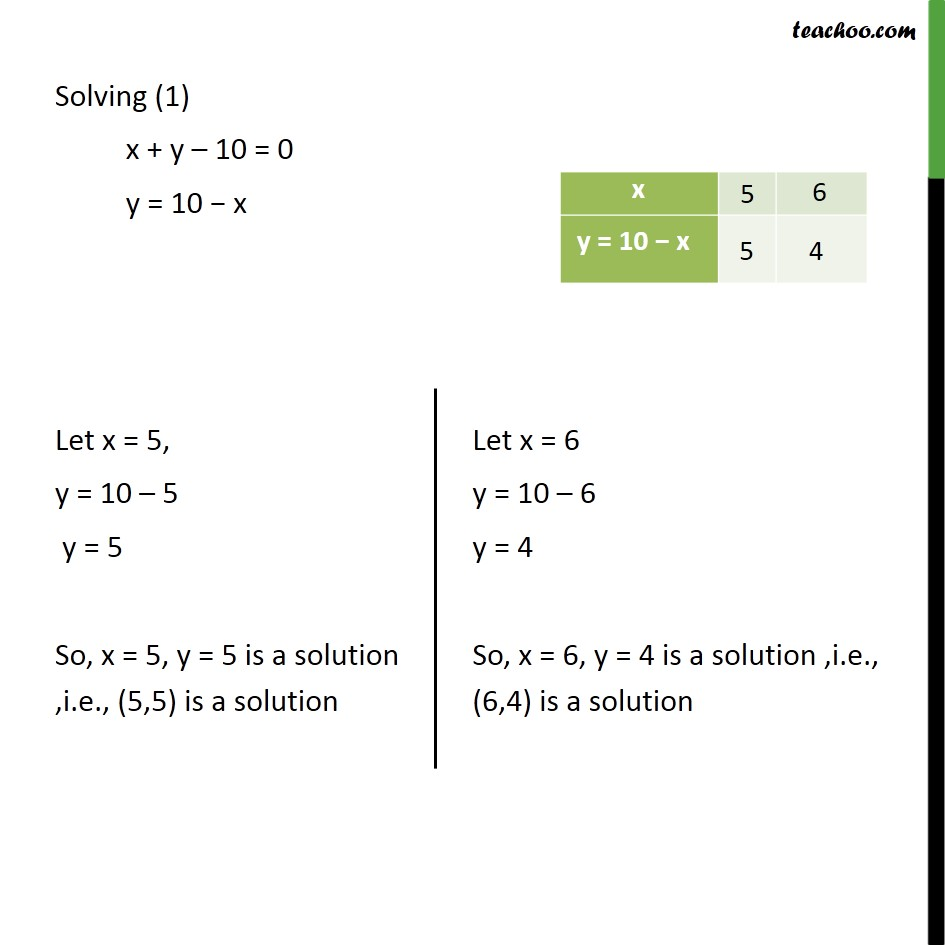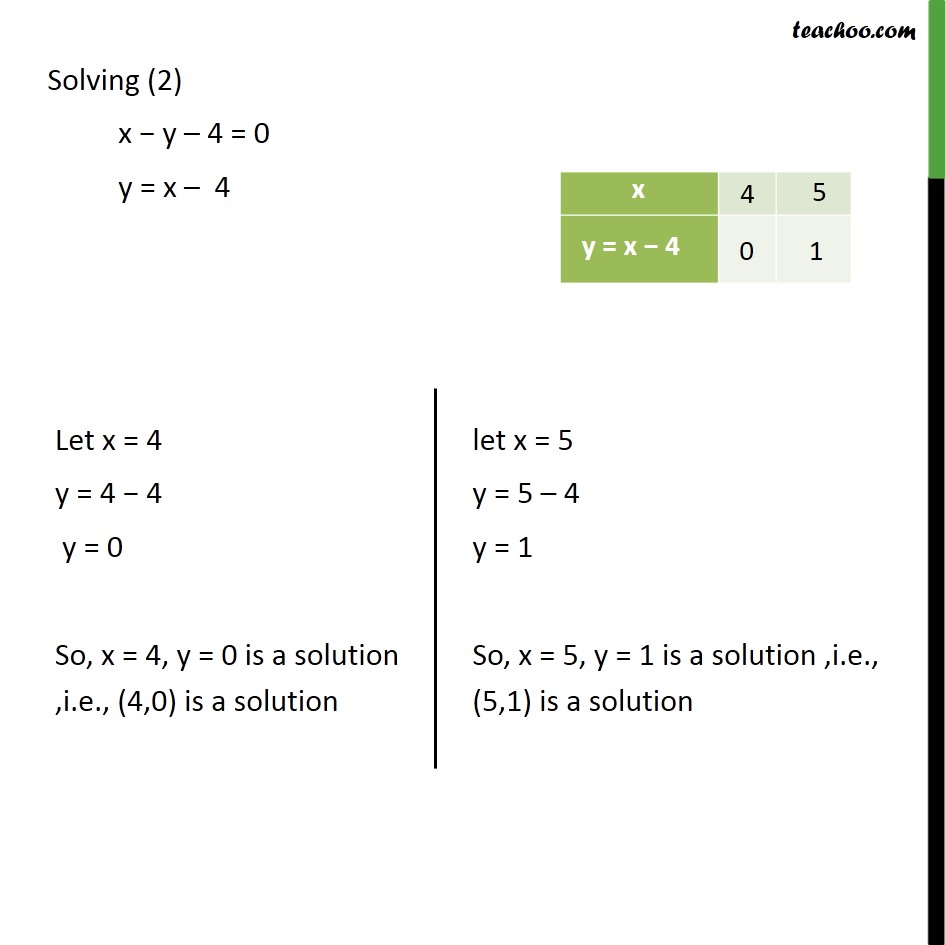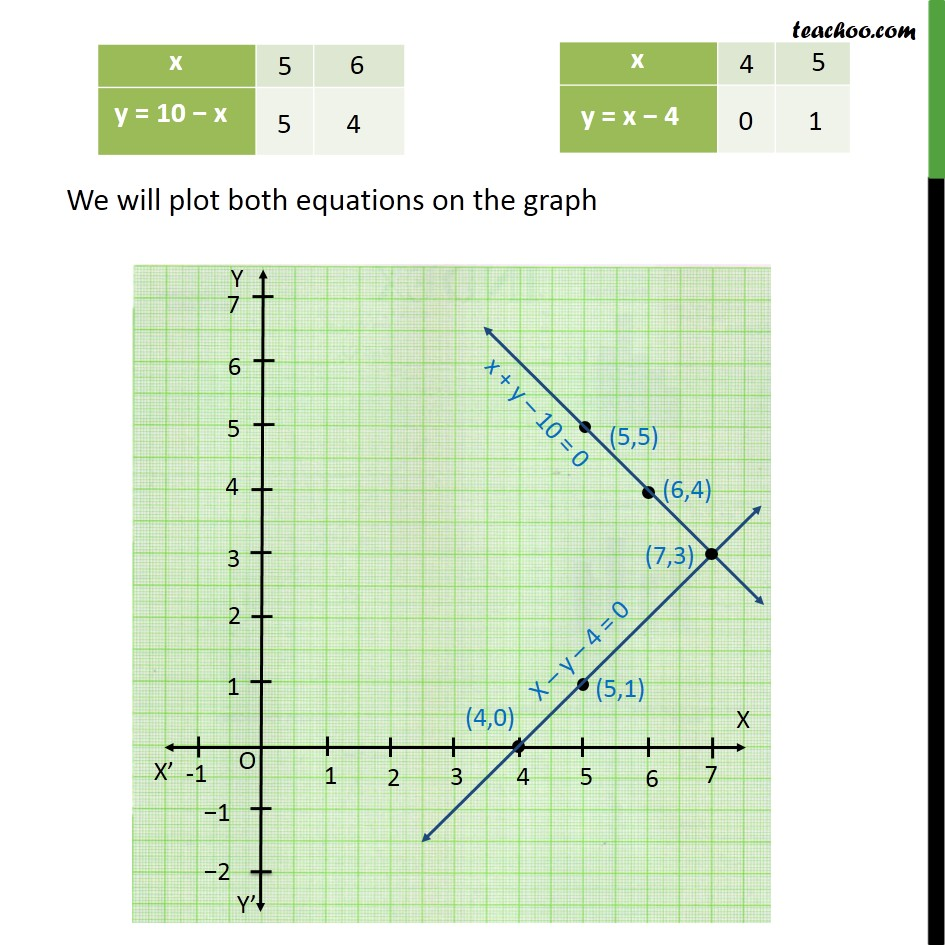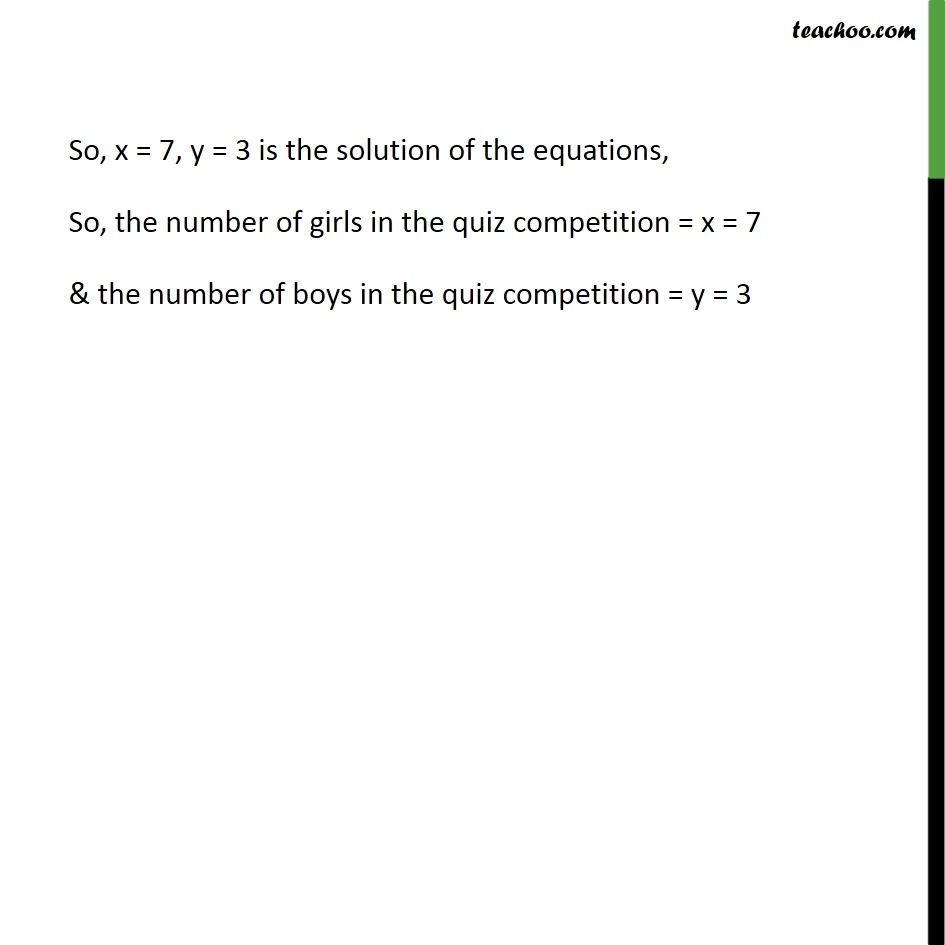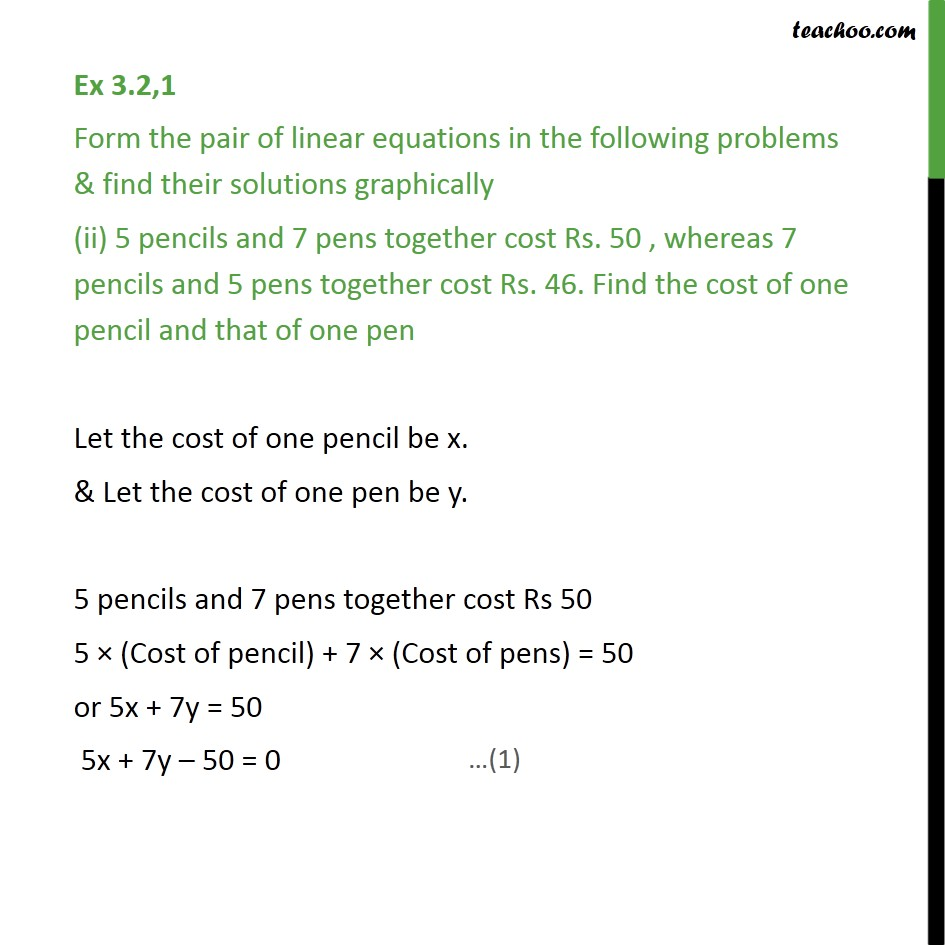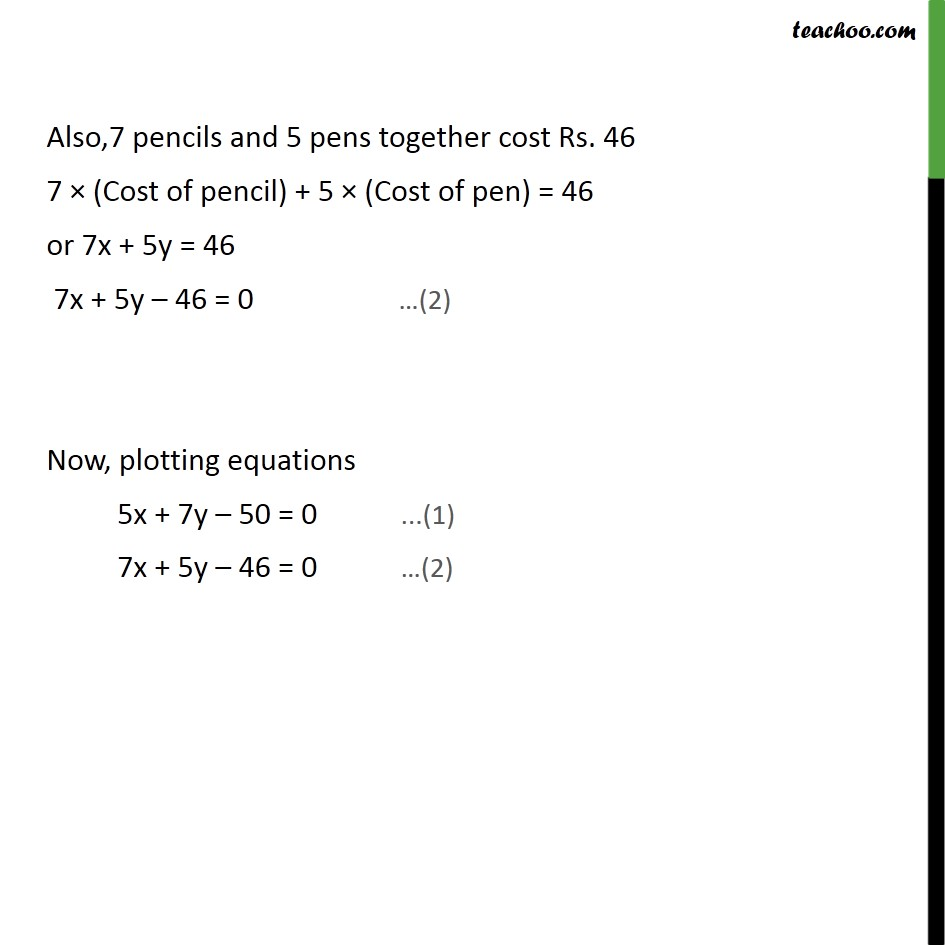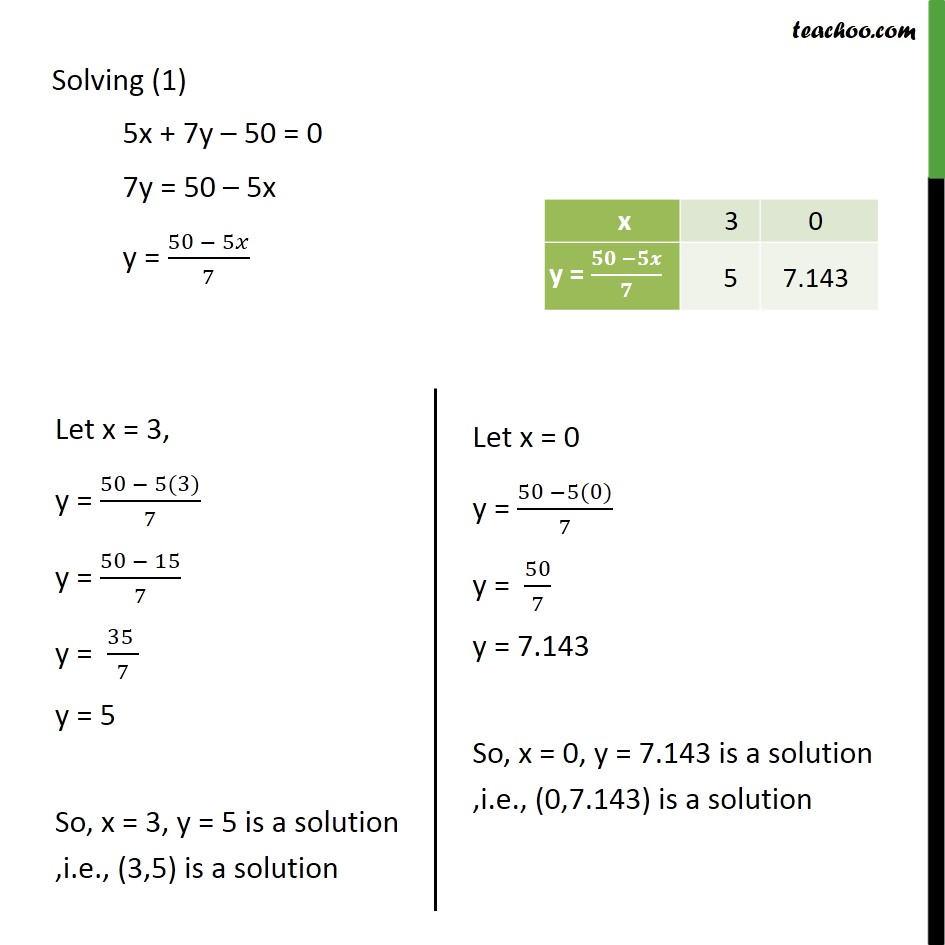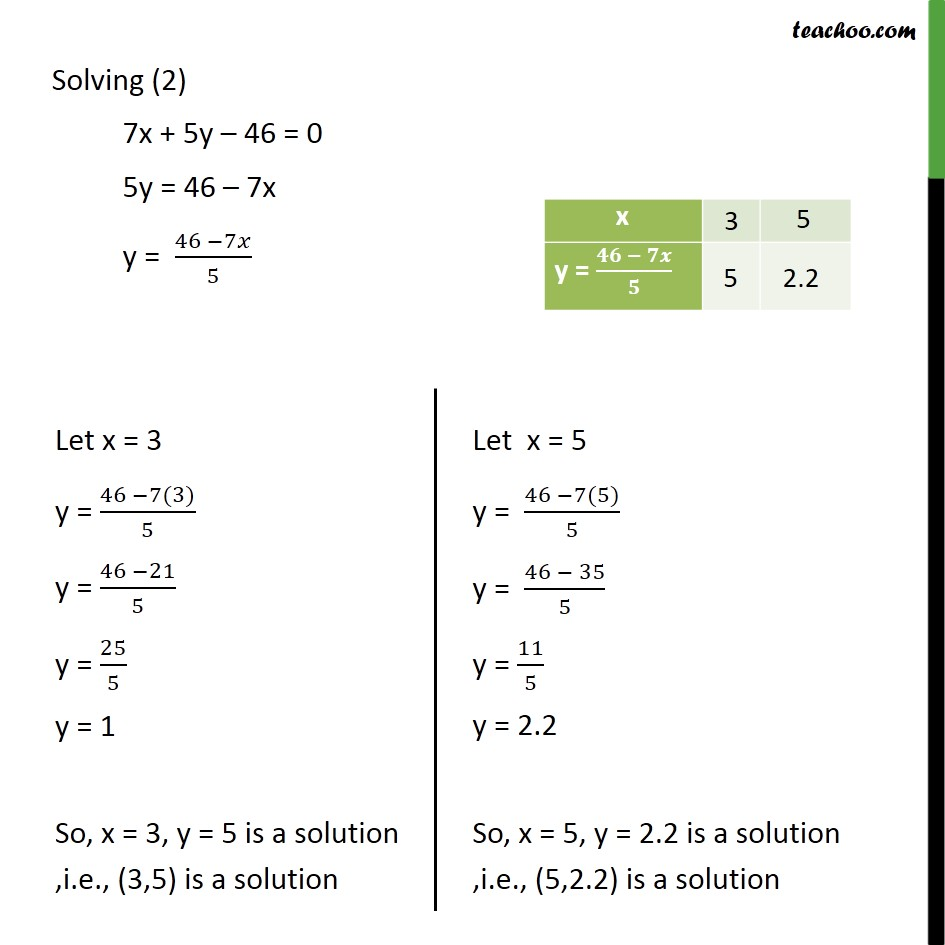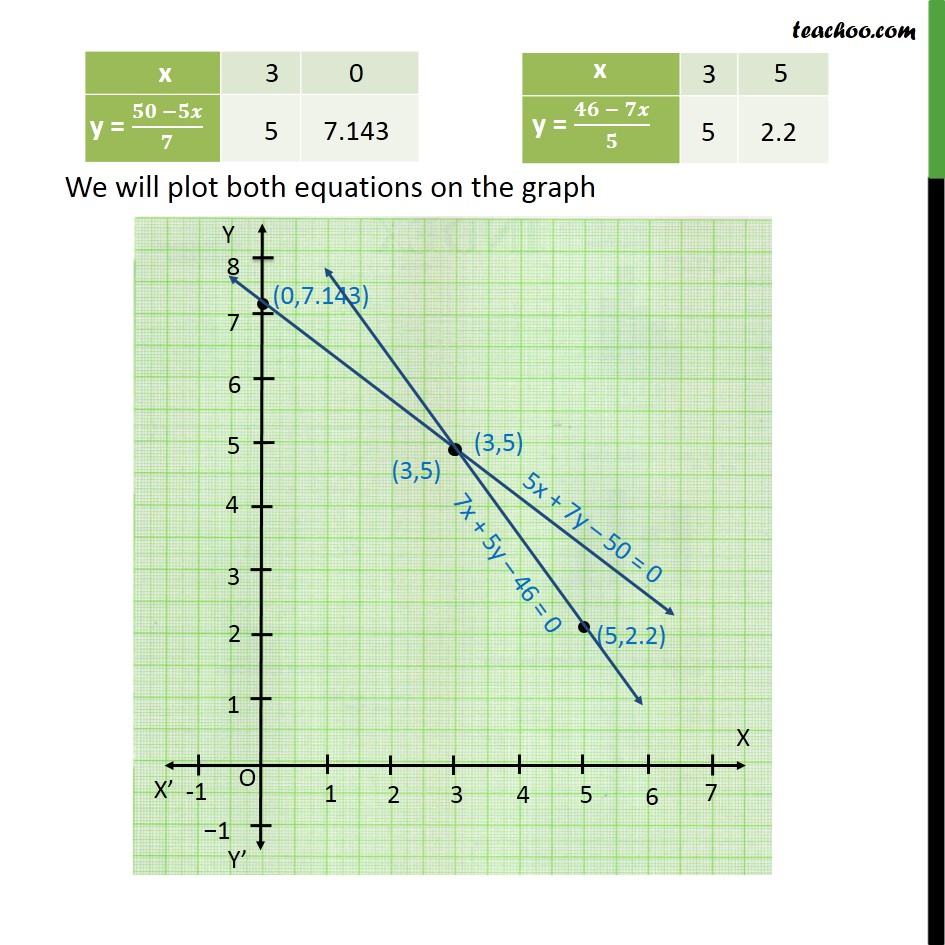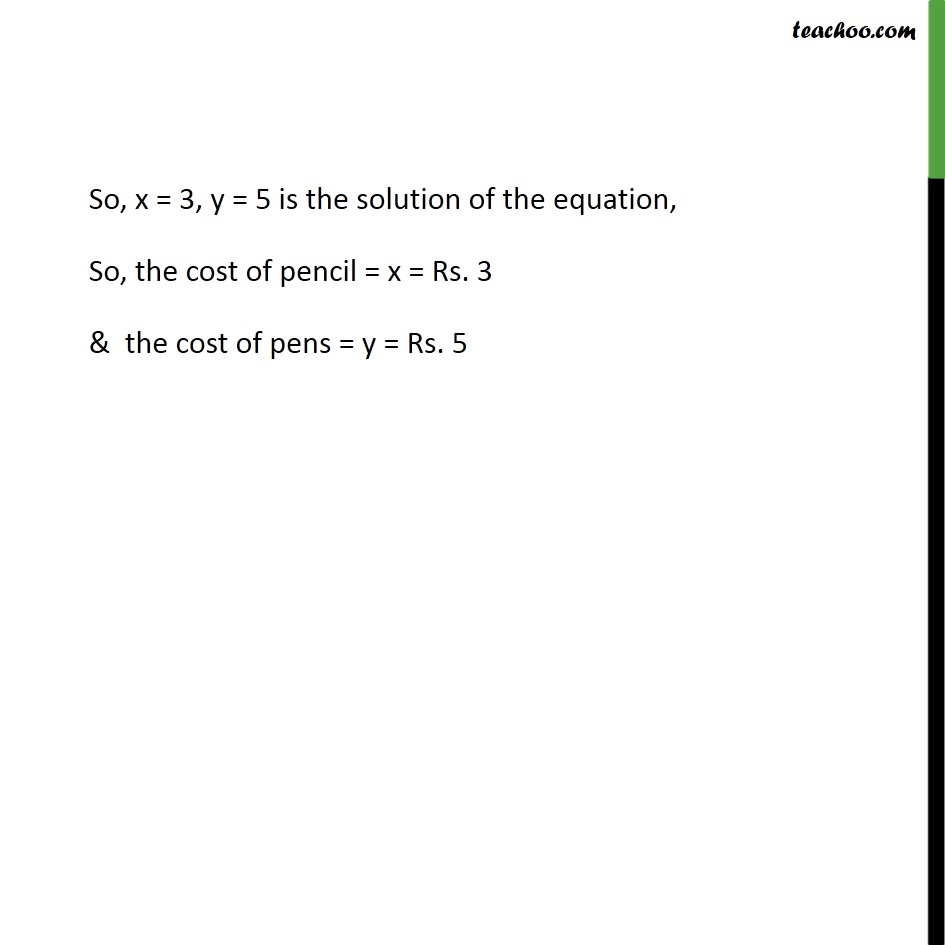1. Chapter 3 Class 10 Pair of Linear Equations in Two Variables
2. Serial order wise
3. Ex 3.2

Transcript

Ex 3.2,1 Form the pair of linear equations in the following problems & find their solutions graphically 10 students of Class X took part in a Mathematics quiz. If the number of girls is 4 more than the number of boys, find the number of boys and girls who took part in the quiz. Let the number of girls who took part in the quiz be x. & Let the number of boys who took part in the quiz be y. Total 10 students took part in the quiz (Number of girls who took part in the quiz) + (Number of boys who took part in the quiz) x + y = 10 x + y – 10 = 0 Also, number of girls is 4 more than boys Number of girls = 4 + Number of boys or x = 4 + y x – y − 4 = 0 Now, plotting equations x + y −10 = 0 ...(1) x − y – 4 = 0 …(2) Solving (1) x + y – 10 = 0 y = 10 − x Solving (2) x − y – 4 = 0 y = x – 4 Ex 3.2,1 Form the pair of linear equations in the following problems & find their solutions graphically (ii) 5 pencils and 7 pens together cost Rs. 50 , whereas 7 pencils and 5 pens together cost Rs. 46. Find the cost of one pencil and that of one pen Let the cost of one pencil be x. & Let the cost of one pen be y. 5 pencils and 7 pens together cost Rs 50 5 × (Cost of pencil) + 7 × (Cost of pens) = 50 or 5x + 7y = 50 5x + 7y – 50 = 0 Also,7 pencils and 5 pens together cost Rs. 46 7 × (Cost of pencil) + 5 × (Cost of pen) = 46 or 7x + 5y = 46 7x + 5y – 46 = 0 Now, plotting equations 5x + 7y – 50 = 0 ...(1) 7x + 5y – 46 = 0 …(2) Solving (1) 5x + 7y – 50 = 0 7y = 50 – 5x y = (50 − 5𝑥)/7 Solving (2) 7x + 5y – 46 = 0 5y = 46 – 7x y = (46 −7𝑥)/5

Ex 3.2